## Modelling Rotationally Symmetric Irregularity with the API

Category: Product News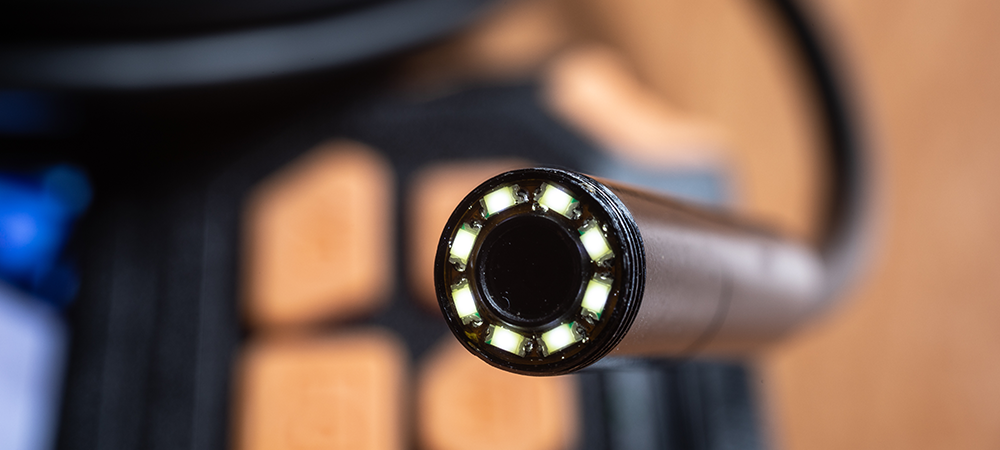In this post we’ll show how the power of the Zemax Application Programming Interface (ZOS-API) with Matlab can be used to model critical manufacturing defects, such Rotationally Symmetric Irregularity (RSI) on a parabolic mirror. Rotationally Symmetric Irregularity (RSI) refers to a group of rotationally symmetric errors in the shape of an optical surface. The errors are represented by Zernike polynomials and have the form of third-order spherical aberration and higher orders of spherical aberration.

Parabolic mirror example

We’ll demonstrate adding RSI and total irregularity to a parabolic mirror.  The mirror is F/3, with an effective focal length of 150 mm.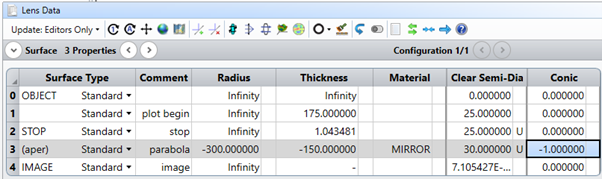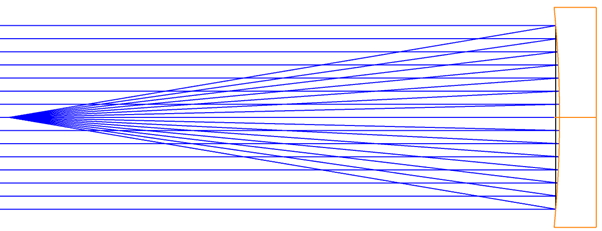For the demonstration, we will add total irregularity and RSI to the surface per this specification:(See Introduction to Rotationally Symmetric Irregularity (RSI) – Knowledgebase (zemax.com) for more details of this specification.)

This will place 1 wave P-V of total irregularity on the surface, and 0.3 waves P-V of RSI.  In nanometers, that will be 500 nm of total surface irregularity, and 150 nm of total RSI.

Structure of the code

We’ve created example Matlab code to model RSI.  This code includes functions that are relevant to modeling irregularity and RSI. The function prepare surface changes a Standard or Even Asphere surface into a Zernike Standard Sag surface. Also included  are several basic supporting functions, such as displayLDE for displaying information from the Lens Data Editor (LDE) of the.ZMX file.

The most important function is AddBC (surface number, wavelength, B, C), where B is the maximum total surface irregularity, and C is the maximum allowed RSI. The AddBC functions work as follows:

• Assigns random values to the RSI terms: Z11, Z22, Z37, and Z56.

• Checks the RMS surface error.

• Scales to achieve the correct value for C.

• This takes just a single scaling because scaling each Zernike coefficient by a constant scales the RMS by the same constant.

• Assigns random values to other Zernike polynomials to model B, total irregularity.

• Without disturbing the values of the RSI terms: Z11, Z22, Z37, and Z56.

• Uses Z5 – Z37 to simulate the total irregularity.

• Iteratively scales the Zernike terms to reach the correct total irregularity.

Running the Matlab example in Interactive Mode

Interactive mode with Matlab and the API is convenient because you can control the API from the Matlab command line. This allows you to try out new commands and change commands on the fly.

You can connect in Interactive mode from the Programming tab by choosing Matlab and then Interactive Extension, as shown below.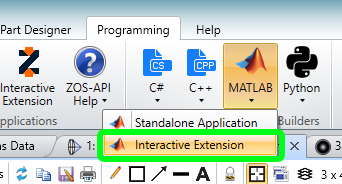The connection code will automatically open.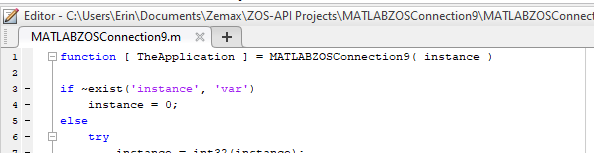Back in OpticStudio, clickling “Interactive Extension” on the Programming tab will put OpticStudio into “listening” mode.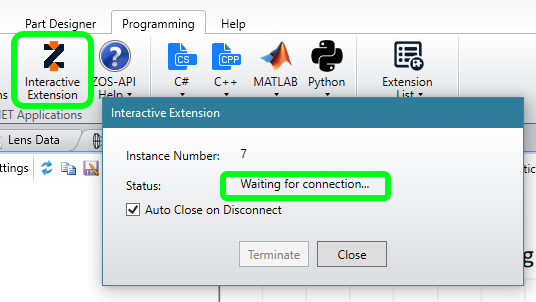In Matlab, now we can connect using the command:

>> TheApplication = MATLABZOSConnection9(7)

Note that your MATLABZOSConnection number and instance may be different than the ones given here.  For example, if this is the first time you’ve connected, you might have MATLABZOSConnection(1).Modeling RSI using Matlab

After making the connection, we can load the file and collect information from our .ZMX file as follows, from the Matlab command line:

>> primary system = TheApplication.PrimarySystem
>> theLDE = primarySystem.LDE
>> theMFE = primarySystem.MFE
>> systemData = primarySystem.SystemData
>> savefilename = System.String.Concat(pwd,'\LensFileForTesting.zmx')
>> primarySystem.SaveAs(savefilename)

All the required functions are contained in the file ErinsABCFunctions.m. By opening this in Matlab we can load the individual functions.

>> abc = ErinsABCFunctions

We prepare the surface by changing it to a Zernike Standard Sag surface.

>> ldetable = abc.displayLDE(primarySystem); disp(ldetable);
>> surfacetable = abc.prepareSurface(primarySystem,3); disp(surfacetable);

The outputs from the above two commands are show below.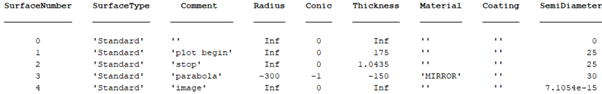For later, we collect the starting sag of the mirror.

>> startingsag = abc.getSag(primarySystem,3);
>> figure(); imagesc(startingsag); axis square;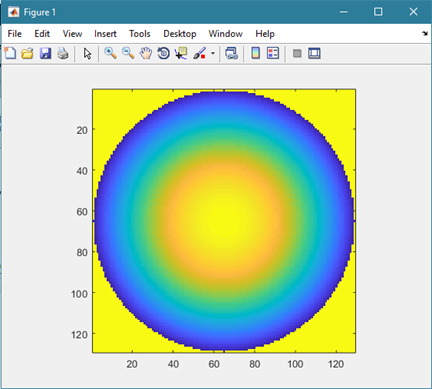Now we can add the B and C surface irregularities using AddBC.

>> bctable = abc.AddBC( primarySystem, 3, 500.0, 1, 0.3);
>> disp( bctable);Note that at this point, if we were carrying out a tolerancing run, we could select random values for B and C when calling AddBC. Collect the perturbed sag of the mirror, and subtract the starting sag so that the surface error perturbations are visible.

>> bcsag = abc.getSag( primarySystem, 3);
>> sagdiff = bcsag-startingsag;
>> figure(); imagesc( sagdiff ); axis square;And we can check the final P-V of these surface errors, too.

>> abs(max( sagdiff(:))-min( sagdiff(:)) / (500*10^-6))

The value is 0.995, rather than the B = 1.000 that we requested. That is due to the accuracy setting used during the iteration needed to scale the Zernikes correctly. A tighter accuracy setting would return an answer closer to 1.000 but would take longer to calculate.

After completing the simulations, we close interactive mode.

>> TheApplication.CloseApplication()

To access the example code discussed in this post, please view the full Knowledgebase article Modeling Rotationally Symmetric Irregularity (RSI) with the API – Knowledgebase (zemax.com).